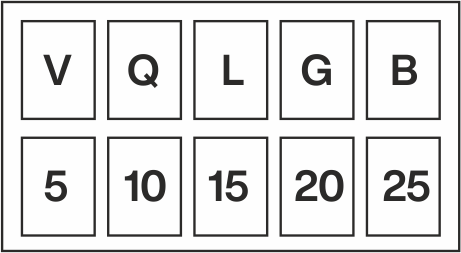Suppose we want to find the place of the 15th letter from the left side. Since now you know that the 15th letter is O, you now need to find a letter which is two positions ahead of O, which is Q.
II. VQLGB: This trick is similar to EJOTY, the only difference being, this is in reverse order i.e. from right to left.## ANALOGOUS PAIR:

Such questions define the relationship between a pair. Here, the first element of the second pair is given, and we have to find the second element of second pair based on similar relationship given by first pair.

Here are a few tips, which would help in answering analogy questions.

It is crucial to understand the relationships among the words and their particular order.

If you make a relationship between the words in a simple sentence, it will be easier for you as the words in the answer will also share a similar analogy.

If two choices are given and they appear to be correct, narrow your approach. Try to elaborate on the meaning / definition in order to arrive at the correct analogy.

Opposites are used to establish an analogy such as. Hot : Cold, Up : Down, Wet : Dry, Brave :Timid, etc.

Objects when put together form a group and so are called Group Analogies.
For example: Soldier-Army, Tree-Forest.

Rhyming Words – Rhyming words are used to establish an analogy - e.g. goat and boat, float and coat, house and mouse etc.

Related Objects - This includes analogy words related to one another e.g. teacher and student, aeroplane and pilot, author and book.

## CLASSIFICATION

Classification means assigning a particular characteristic to various members / part of a group, and further identifying them on the basis of that particular characteristic only.
Example :
Choose the word which is least like the others word in a group.
A. Apple B. Grapes C. Banana D. Onion E. Kiwi
Explanation: All the other are fruits, while onion is a vegetable.

## CODING AND DECODING

Tips:
Observe the letters or numbers given in the code thoroughly. Find the sequence it follows, ascending or descending.

Find the rule in which the letters / numbers / words follow. Fill the appropriate letter / number / word in the given blank.

Letter position (A = 1, B = 2, C = 3, D = 4 ……… Y = 25, Z = 26)
Opposite position of letters (A = 26, B = 25 ……. Z = 1)
Opposite of each letter (A is opposite to Z and B is opposite to Y and C is opposite to X ....... and so on)

## LETTER CODING

Letter coding involves the real letters in a word being replaced by other letters according to a specific rule, to form its code.

SUBSTITUTION:

The name of an object is substituted with different names of the object.

MIXED LETTER CODING:

We take any two messages with a common word to analyse such codes. The common code word will be the meaning of that word. Therefore, by picking up all possible combinations of two, the entire message can be analysed.